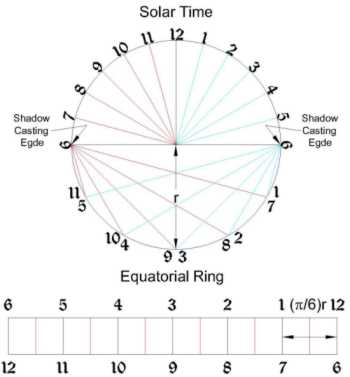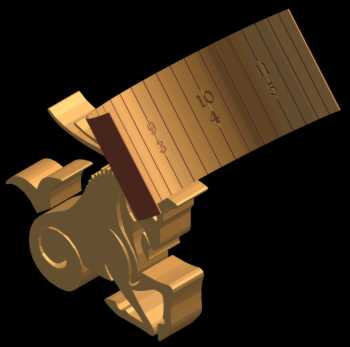The Sundial Primer created by Carl SabanskiThe Sundial Primer Index
 Gnomon-less Equatorial Sundial Equatorial Sundial: a dial in which the dial plate is parallel to the equatorial plane and the polar-pointing gnomon is perpendicular to it.Equatorial Plane: the plane through the Earth defined by the equator. The equatorial sundial described here is similar to the equatorial ring sundial. It does not have a gnomon but uses the two edges of the equatorial ring as the shadow casting edges or styles.Figure 1 illustrates the template for a gnomon-less equatorial dial. The full hour lines are spaced at 30° intervals around the ring. The angle of intermediate hour lines can be easily calculated. Each hour line represents two times of the day, one in the morning and the other in the afternoon.  One style or shadow casting edge will indicate the morning hours and the other the afternoon hours.Figure 1: Gnomon-less Equatorial Sundial Template (CAD) If the ring is laid out as a flat rectangular plate, the hour lines are equally spaced apart. Knowing the inside radius "r" of the desired ring dimension the distance between full hour lines is given by (p /6)r. The distance to intermediate hour lines can also be calculated. Once the hour lines and numbers are inscribed the plate can be bent to the correct diameter. Note that you are actually looking at the back of the plate in Figure 1 and the mirror image must be used when laying out the actual sundial. To ensure that the shadow will always fall on the dial plate throughout the year the ring width is determined as follows:w = tan (23.44°) x dw = 0.4336 x dwhere d = 2 x r and is the diameter of the equatorial sundial ring. For the gnomon-less equatorial dial to be used at any latitude the user must only ensure that the styles point to the celestial pole. To do this the dial plate must be positioned so that it is at an angle with a horizontal surface equal to the latitude. This sundial can be adjusted for the Equation of Time and longitude correction by rotating the dial plate around either style. As the shadow moves up the dial plate and approaches either style it becomes more difficult to read.For an image complete with shadow click here.